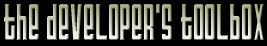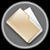This section of the archives stores flipcode's complete Developer Toolbox collection, featuring a variety of mini-articles and source code contributions from our readers.Plug n' Play Collision Detection For Spheres   Submitted byThis is "theoretically" a plug and play collision detection routine that anyone can include in their programs. Practically, it's plug and play if you're using DirectX8 and Microsoft Visual Studio, since that's what I used.

However, it should be fairly easy to go through and change any D3DXVECTOR3 typedefs to your own desired format.

The class is very simple to use. Simply declare a CCollide object wherever you want. You could declare a global one, but since it keeps a permanent record of the last "safe" position, it is recommended that you encapsulate this class in whatever class you are using.

This class is neither optimized nor perfect, and is intended to be used as a gateway for people who just want to get something up and running, with the intention of coming back to it later. I welcome any recommended changes or advice. The class is based on Telemacho's collision detection routines, and he deserves full credit for it. I only encapsulated it so a person can essentially plug it in and use it. I also made a few small changes to correct some errors of missed collisions, on the advice of BigB on this very message board.

Just include Collide.cpp and Collide.h in your project, and you should be ready to go.

 ```To define:CCollide TheCollision;To use:D3DXVECTOR3 initialposition; // Start position of the object you want to move D3DXVECTOR3 movementvector; // Direction and speed to move it in D3DXVECTOR3 finalposition; // Final position where object "should" be float radiusofsphere; // Radius of the bounding sphere for the object finalposition=TheCollision.Move(initialposition,movementvector,radiusofsphere) ```

Finalposition will be filled with the correct position of the object, taking all collisions and sliding into consideration.
Some limitations:

Currently browsing [collide.zip] (4,514 bytes) - [Collide.cpp] - (12,108 bytes)

 ```// Collide.cpp: implementation of the CCollide class. // ////////////////////////////////////////////////////////////////////// #include "stdafx.h" #include "Collide.h"////////////////////////////////////////////////////////////////////// // Construction/Destruction ////////////////////////////////////////////////////////////////////// //#define EPSILON 0.015f //#define SECONDARYEPSILON 0.055f inline float dot(D3DXVECTOR3& v1, D3DXVECTOR3& v2) { return ( v1.x * v2.x + v1.y * v2.y + v1.z * v2.z ); }inline float lengthOfVector(D3DXVECTOR3 v) { return (float)(sqrt(v.x * v.x + v.y * v.y + v.z * v.z)); }inline void setLength(D3DXVECTOR3& v, float l) { float len = (float)sqrt(v.x*v.x + v.y*v.y + v.z*v.z); v.x *= l/len; v.y *= l/len; v.z *= l/len; } inline BOOL isZeroVector(D3DXVECTOR3& v) { if ((v.x == 0.0f) && (v.y == 0.0f) && (v.z == 0.0f)) return TRUE; return FALSE; }inline void normalizeVector(D3DXVECTOR3& v) { float len = (float)sqrt(v.x*v.x + v.y*v.y + v.z*v.z); v.x /= len; v.y /= len; v.z /= len; }inline D3DXVECTOR3 wedge(D3DXVECTOR3 v1, D3DXVECTOR3 v2) { D3DVECTOR result; result.x = (v1.y * v2.z) - (v2.y * v1.z); result.y = (v1.z * v2.x) - (v2.z * v1.x); result.z = (v1.x * v2.y) - (v2.x * v1.y); return result; }#define PLANE_BACKSIDE 0x000001 #define PLANE_FRONT 0x000010 #define ON_PLANE 0x000100DWORD classifyPoint(D3DXVECTOR3 point, D3DXVECTOR3 pO, D3DXVECTOR3 pN) { D3DXVECTOR3 dir = pO - point; float d=dot(dir,pN); if (d<-0.001f) return PLANE_FRONT; else if (d>0.001f) return PLANE_BACKSIDE; return ON_PLANE; }float intersectRaySphere(D3DXVECTOR3 rO, D3DXVECTOR3 rV, D3DXVECTOR3 sO, float sR) { D3DXVECTOR3 Q = sO-rO; float c = lengthOfVector(Q); float v =dot(Q,rV); float d = sR*sR - (c*c - v*v); // If there was no intersection, return -1 if (d < 0.0) return (-1.0f); // Return the distance to the [first] intersecting point return (v - (float)sqrt(d)); }BOOL CheckPointInTriangle(D3DXVECTOR3 point, D3DXVECTOR3 a, D3DXVECTOR3 b, D3DXVECTOR3 c) { float total_angles = 0.0f; // make the 3 vectors D3DXVECTOR3 v1 = point-a; D3DXVECTOR3 v2 = point-b; D3DXVECTOR3 v3 = point-c; normalizeVector(v1); normalizeVector(v2); normalizeVector(v3); total_angles += (float)acos(dot(v1,v2)); total_angles += (float)acos(dot(v2,v3)); total_angles += (float)acos(dot(v3,v1)); int u = _finite(total_angles); if( (fabs(total_angles-2*PI) <= 0.005)&&(u==1) ) //if (fabs(total_angles-2*PI) <= 0.005) return (TRUE); return(FALSE); }D3DXVECTOR3 closestPointOnLine(D3DXVECTOR3& a, D3DXVECTOR3& b, D3DXVECTOR3& p) { // Determine t (the length of the vector from �a� to �p�) D3DXVECTOR3 c = p-a; D3DXVECTOR3 V = b-a; float d = lengthOfVector(V); normalizeVector(V); float t = dot(V,c); if (t < 0.0f) return (a); if (t > d) return (b); V.x = V.x * (float)t; V.y = V.y * (float)t; V.z = V.z * (float)t; return (a+V); }D3DVECTOR closestPointOnTriangle(D3DXVECTOR3 a, D3DXVECTOR3 b, D3DXVECTOR3 c, D3DXVECTOR3 p) { D3DXVECTOR3 Rab = closestPointOnLine(a, b, p); D3DXVECTOR3 Rbc = closestPointOnLine(b, c, p); D3DXVECTOR3 Rca = closestPointOnLine(c, a, p); D3DXVECTOR3 pRab=p-Rab; D3DXVECTOR3 pRbc=p-Rbc; D3DXVECTOR3 pRca=p-Rca; float dAB = lengthOfVector(pRab); float dBC = lengthOfVector(pRbc); float dCA = lengthOfVector(pRca); float min = dAB; D3DXVECTOR3 result = Rab; if (dBC < min) { min = dBC; result = Rbc; } if (dCA < min) result = Rca; return (result); }BOOL CheckPointInSphere(D3DXVECTOR3 point, D3DXVECTOR3 sO, float sR) { D3DXVECTOR3 pointsO=point-sO; float d = lengthOfVector(pointsO); if(d<= sR) return TRUE; return FALSE; }void SetVectorLength(D3DVECTOR& v, float l) { float len = (float)sqrt(v.x*v.x + v.y*v.y + v.z*v.z); v.x *= l/len; v.y *= l/len; v.z *= l/len; } CCollide::CCollide() { EPSILON=0.5f; SECONDARYEPSILON=0.5f; }CCollide::~CCollide() { } float intersectRayPlane(D3DXVECTOR3 rOrigin, D3DXVECTOR3 rVector, D3DXVECTOR3 pOrigin, D3DXVECTOR3 pNormal) { float d = - (dot(pNormal,pOrigin)); float numer = dot(pNormal,rOrigin) + d; float denom = dot(pNormal,rVector); if (denom == 0) // normal is orthogonal to vector, cant intersect return (-1.0f); return -(numer / denom); } D3DXVECTOR3 CCollide::Move(D3DXVECTOR3 Position, D3DXVECTOR3 Velocity, float Radius) { D3DXVECTOR3 scaledPosition, scaledVelocity; D3DXVECTOR3 Result; scaledPosition = Position/(Radius); scaledVelocity = Velocity/(Radius); Result=CollideWithWorld(scaledPosition, scaledVelocity,Radius); Result=Result*(Radius); return Result; } D3DXVECTOR3 CCollide::CollideWithWorld(D3DXVECTOR3 position, D3DXVECTOR3 velocity, float Radius) { D3DXVECTOR3 pos; if (lengthOfVector(velocity)=EPSILON) { D3DXVECTOR3 V=velocity; SetVectorLength(V,(float)collision.nearestDistance-SECONDARYEPSILON); newSourcePoint=collision.sourcePoint+V; } else { newSourcePoint = collision.sourcePoint; } D3DVECTOR slidePlaneOrigin=collision.nearestPolygonIntersectionPoint; D3DVECTOR slidePlaneNormal=newSourcePoint-collision.nearestPolygonIntersectionPoint; float l=intersectRayPlane(destinationPoint,slidePlaneNormal,slidePlaneOrigin,slidePlaneNormal); D3DXVECTOR3 newDestinationPoint; newDestinationPoint.x = (float)(destinationPoint.x + l * slidePlaneNormal.x); newDestinationPoint.y = (float)(destinationPoint.y + l * slidePlaneNormal.y); newDestinationPoint.z = (float)(destinationPoint.z + l * slidePlaneNormal.z); // Generate the slide vector, which will become our new velocity vector // for the next iteration D3DVECTOR newVelocityVector = newDestinationPoint - collision.nearestPolygonIntersectionPoint; // now we recursively call the function with the new position and velocity collision.lastSafePosition = position; return CollideWithWorld(newSourcePoint, newVelocityVector,Radius); return position; } }void CCollide::CheckCollision(TCollisionPacket* colPackage) { D3DXVECTOR3 p1,p2,p3; D3DXVECTOR3 pNormal; D3DXVECTOR3 pOrigin; D3DXVECTOR3 v1, v2; D3DXVECTOR3 source = colPackage->sourcePoint; D3DXVECTOR3 eRadius = colPackage->eRadius; D3DXVECTOR3 velocity = colPackage->velocity; D3DXVECTOR3 normalizedVelocity = velocity; normalizeVector(normalizedVelocity); D3DXVECTOR3 sIPoint; // sphere intersection point D3DXVECTOR3 pIPoint; // plane intersection point D3DXVECTOR3 polyIPoint; // polygon intersection point double distanceToTravel=lengthOfVector(velocity); double distToPlaneIntersection; double distToEllipsoidIntersection; // Okay, now this is a test to go through all the triangles. short scan_triangle; LPTRIANGLE tri; for (scan_triangle=0;scan_triangletriangles+scan_triangle); if (tri==NULL) break; p1.x=tri->v.x/eRadius.x; p1.y=tri->v.y/eRadius.y; p1.z=tri->v.z/eRadius.z; p2.x=tri->v.x/eRadius.x; p2.y=tri->v.y/eRadius.y; p2.z=tri->v.z/eRadius.z; p3.x=tri->v.x/eRadius.x; p3.y=tri->v.y/eRadius.y; p3.z=tri->v.z/eRadius.z; pOrigin = p1; v1= p2-p1; v2= p3-p1; pNormal = wedge(v1, v2); normalizeVector(pNormal); if (dot(pNormal,normalizedVelocity) <= 1.0f) { // calculate sphere intersection point sIPoint = source - pNormal; // classify point to determine if ellipsoid span the plane DWORD pClass = classifyPoint(sIPoint, pOrigin, pNormal); if (pClass==PLANE_BACKSIDE) { // find plane intersection point by shooting a ray from the // sphere intersection point along the planes normal. distToPlaneIntersection = intersectRayPlane(sIPoint, pNormal, pOrigin, pNormal); // calculate plane intersection point pIPoint.x = sIPoint.x + (float)distToPlaneIntersection * pNormal.x; pIPoint.y = sIPoint.y + (float)distToPlaneIntersection * pNormal.y; pIPoint.z = sIPoint.z + (float)distToPlaneIntersection * pNormal.z; } else { // shoot ray along the velocity vector distToPlaneIntersection = intersectRayPlane(sIPoint, normalizedVelocity, pOrigin, pNormal); // calculate plane intersection point pIPoint.x = sIPoint.x + (float)distToPlaneIntersection * normalizedVelocity.x; pIPoint.y = sIPoint.y + (float)distToPlaneIntersection * normalizedVelocity.y; pIPoint.z = sIPoint.z + (float)distToPlaneIntersection * normalizedVelocity.z; } // find polygon intersection point. By default we assume its equal to the // plane intersection point polyIPoint = pIPoint; distToEllipsoidIntersection = distToPlaneIntersection; if (!CheckPointInTriangle(pIPoint,p1,p2,p3)) { // if not in triangle polyIPoint = closestPointOnTriangle(p1, p2, p3, pIPoint); distToEllipsoidIntersection = intersectRaySphere(polyIPoint, -normalizedVelocity, source, 1.0f); if (distToEllipsoidIntersection > 0) { // calculate true sphere intersection point sIPoint.x = polyIPoint.x + (float)distToEllipsoidIntersection * -normalizedVelocity.x; sIPoint.y = polyIPoint.y + (float)distToEllipsoidIntersection * -normalizedVelocity.y; sIPoint.z = polyIPoint.z + (float)distToEllipsoidIntersection * -normalizedVelocity.z; } } if (CheckPointInSphere(polyIPoint, source, 1.0f)) { //if (this->tag==TRUE) tri->flag=2; colPackage->stuck=TRUE; } if ((distToEllipsoidIntersection > 0) && (distToEllipsoidIntersection <= distanceToTravel)) { if ((colPackage->foundCollision == FALSE) || (distToEllipsoidIntersection < colPackage->nearestDistance)) { //if (this->tag==TRUE) tri->flag=1; colPackage->nearestDistance = (float)distToEllipsoidIntersection; colPackage->nearestIntersectionPoint = sIPoint; colPackage->nearestPolygonIntersectionPoint = polyIPoint; colPackage->foundCollision = TRUE; } } } } }void CCollide::Tweak(float epsilon, float secondaryepsilon) { EPSILON=epsilon; SECONDARYEPSILON=secondaryepsilon; } ```

Currently browsing [collide.zip] (4,514 bytes) - [Collide.h] - (1,917 bytes)

 ```// Collide.h: interface for the CCollide class. // ////////////////////////////////////////////////////////////////////// #if !defined(AFX_COLLIDE_H__E3F57089_A057_4886_8BA2_DD8476C45089__INCLUDED_) #define AFX_COLLIDE_H__E3F57089_A057_4886_8BA2_DD8476C45089__INCLUDED_#if _MSC_VER > 1000 #pragma once #endif // _MSC_VER > 1000 #include #include #include #include #include #include #define PI 3.141592654ftypedef struct _VERTEX { float x, y, z; // position float nx, ny, nz; // normal DWORD Diffuse; // diffuse color float tu1, tv1; // texture coordinates } VERTEX, *LPVERTEX;typedef struct _TRIANGLE { char flag; VERTEX v; } TRIANGLE, *LPTRIANGLE;class CCollide { public: float EPSILON; float SECONDARYEPSILON; BOOL tag; // True to color impacted surfaces LPTRIANGLE *triangles; int triangle_count; struct TCollisionPacket { // data about player movement D3DXVECTOR3 velocity; D3DXVECTOR3 sourcePoint; // radius of ellipsoid. D3DXVECTOR3 eRadius; // for error handling D3DXVECTOR3 lastSafePosition; BOOL stuck; // data for collision response BOOL foundCollision; float nearestDistance; // nearest distance to hit D3DXVECTOR3 nearestIntersectionPoint; // on sphere D3DXVECTOR3 nearestPolygonIntersectionPoint; // on polygon } collision; D3DXVECTOR3 Move(D3DXVECTOR3 Position, D3DXVECTOR3 Velocity, float Radius); D3DXVECTOR3 CollideWithWorld(D3DXVECTOR3 position, D3DXVECTOR3 velocity, float Radius); void CheckCollision(TCollisionPacket* colPackage); void Tweak(float epsilon, float secondaryepsilon); CCollide(); virtual ~CCollide(); };#endif // !defined(AFX_COLLIDE_H__E3F57089_A057_4886_8BA2_DD8476C45089__INCLUDED_) ```

The zip file viewer built into the Developer Toolbox made use of the zlib library, as well as the zlibdll source additions.